Search

Completing the Square: Circle EquationsThe technique of completing the square is used to turn a quadratic into the sum of a squared binomial and a number: (x  a)2 + b. The center-radius form of the circle equation is in the format (x  h)2 + (y  k)2 = r2, with the center being at the point (h, k) and the radius being "r". This form of the equation is helpful, since you can easily find the center and the radius.

But circle equations are often given in the general format of ax2 + by2 + cx + dy + e = 0, When you are given this general form of equation and told to find the center and radius of a circle, you will have to "complete the square" to convert the equation to center-radius form. This lesson explains how to make that conversion. Copyright © Elizabeth Stapel 2000-2011 All Rights Reserved

• Find the center and radius of the circle having the following equation:

4x2 + 4y2  16x  24y + 51 = 0.

 Here is the equation they've given you.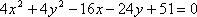Move the loose number over to the other side.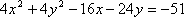Group the x-stuff together. Group the y-stuff together.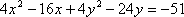Whatever is multiplied on the squared terms (it'll always be the same number), divide it off from every term.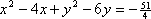This is the complicated step. You'll need space inside your groupings, because this is where you'll add the squaring term. Take the x-term coefficient, multiply it by one-half, square it, and then add this to both sides of the equation, as shown. Do the same with the y-term coefficient.  Convert the left side to squared form, and simplify the right side.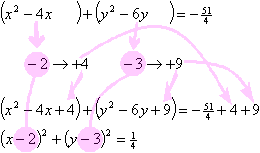Read off the answer from the rearranged equation. The center is at (h, k) = (x, y) = (2, 3). The radius is r = sqrt( 1/4 ) = 1/2

Completing the square to find a circle's center and radius always works in this manner. Always do the steps in this order, and each of your exercises should work out fine. (Also, if you get in the habit of always working the exercises in the same manner, you are more likely to remember the procedure on tests.)

Warning: Don't misinterpret the final equation. Remember that the circle formula is (x  h)2 + (y  k)2 = r2. If you end up with an equation like (x + 4)2 + (y + 5)2 = 5, you have to keep straight that h and k are subtracted in the center-radius form, so you really have (x  (4))2 + (y  (5))2 = 5. That is, the center is at the point (4, 5), not at (4, 5). Be careful with the signs; don't just "read off the answer" without thinking. Also, remember that the formula says "r2", not "r", so the radius in this case is sqrt(5), not 5.

In the course of the above procedure, about the only other thing that can be a problem is forgetting the sign on the step where you multiply by one-half. Warning: If you drop a negative, you'll get the wrong answer for the coordinates of the center, so be careful of this. Don't try to do this step in your head: write it out!

Here's one more example of how completing the square works for circle equations:

• Find the center and radius of the circle with the following equation:

100x2 + 100y2  100x + 240y  56 = 0.

 This is the given equation.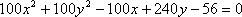Move the loose number over to the other side.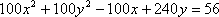Group the x-stuff and y-stuff together.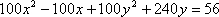Divide off by whatever is multiplied on the squared terms.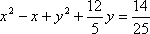Take the coefficient on the x-term, multiply by one-half, square, and add inside the x-stuff and also to the other side. Do the same with the y-term.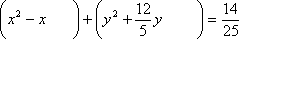Convert the left-hand side to squared form, and simplify the right-hand side.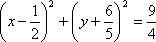If necessary, fiddle with signs and exponents to make your equation match the circle equation's format.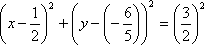Read off the answer. The center is at ( 1/2,  6/5 ) and the radius is 3/2.

 Cite this article as: Stapel, Elizabeth. "Completing the Square: Circle Equations." Purplemath. Available from     https://www.purplemath.com/modules/sqrcircle.htm. Accessed [Date] [Month] 2016

Study Skills Survey

Tutoring from Purplemath
Find a local math tutor